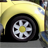# Conditional (if / then / else) logic in Survey123 using numeric answers

9413
4
03-19-2021 12:40 PMNew Contributor II

Hi there,

I'm trying to use conditional if / then / else logic in Survey123 to take a range of numeric values calculated in (question_one) and assign it a value accordingly.

I've tried modifying this script (from: https://doc.arcgis.com/en/survey123/desktop/create-surveys/xlsformformulas.htm#ESRI_SECTION2_BED1A39...😞

 if(condition, a, b) If the condition evaluates to true, returns a; otherwise, returns b. if(selected(\${question_one}, 'yes') and selected(\${question_two}, 'yes'), 'yes', 'no')

The conditional logic would be something like:

if(\${question_one} > 1.0 and < 1.9) then assigned_value = '500mm'

if(\${question_one} > 2.0 and < 2.9) then assigned_value = '600mm'

else(\${question_one} > 2.0 and < 2.9) then assigned_value = '700mm'

I've tried this format (and similar variations):

if(\${Q100} > 0.5), '500'

Note: question_one is a numeric field (decimal) that calculates a total based on other fields in the form (i.e. an average). The assigned_value field would then use that auto-calculated numeric value field and categorize the numeric values based on ranges (i.e. 1.0 to 2.9 or >= 1 and <= 2.9).

Is this possible within Survey123. Does anyone have experience using a similar loop conditional statement?

SJ

Tags (4)
1 Solution

Accepted SolutionsbyMVP Honored Contributor

You are just having some syntax issues.

First you always need to build both sides of an And or Or.

if(\${question_one} > 1.0 and \${question_one} < 1.9, '500mm', 'no')

123 does not have esleif so it does get harder to chain them together.  But it goes like this

if(\${question_one} > 1.0 and \${question_one} < 1.9, '500mm', if(\${question_one} > 2.0 and \${question_one} < 2.9, '600mm', if(\${question_one} > 2.0 and \${question_one} < 2.9, '700mm', 'none of the above')))

So same logic, it just needs to be written on all one line. if(condition, true, false)  Hope that helps.

4 RepliesbyMVP Honored Contributor

You are just having some syntax issues.

First you always need to build both sides of an And or Or.

if(\${question_one} > 1.0 and \${question_one} < 1.9, '500mm', 'no')

123 does not have esleif so it does get harder to chain them together.  But it goes like this

if(\${question_one} > 1.0 and \${question_one} < 1.9, '500mm', if(\${question_one} > 2.0 and \${question_one} < 2.9, '600mm', if(\${question_one} > 2.0 and \${question_one} < 2.9, '700mm', 'none of the above')))

So same logic, it just needs to be written on all one line. if(condition, true, false)  Hope that helps.New Contributor II

Thank you! This worked out perfect! 🙂New Contributor

Dear colleagues,

I am struggling with very simple question. Please help to sort i out. I have a string quesion {crop} with dropdown and one choice should be selected. Have another string quesion {alt_crop} to polulate based one choice from {crop}.

Struggling with syntaxis here (not working):

if(selected(\${crop},'Corn'), 'Winter wheat', if(selected(\${crop},'Winter wheat'), 'Sunflower', 'na'))

Survey is done based on existing layer ({crop} haas domains

what is wrong here?Occasional Contributor III

What is happening when you try to save/run the calculation? The first thing that comes to mind is use the domain values, not the display names as I was encountering this earlier with a colleague.